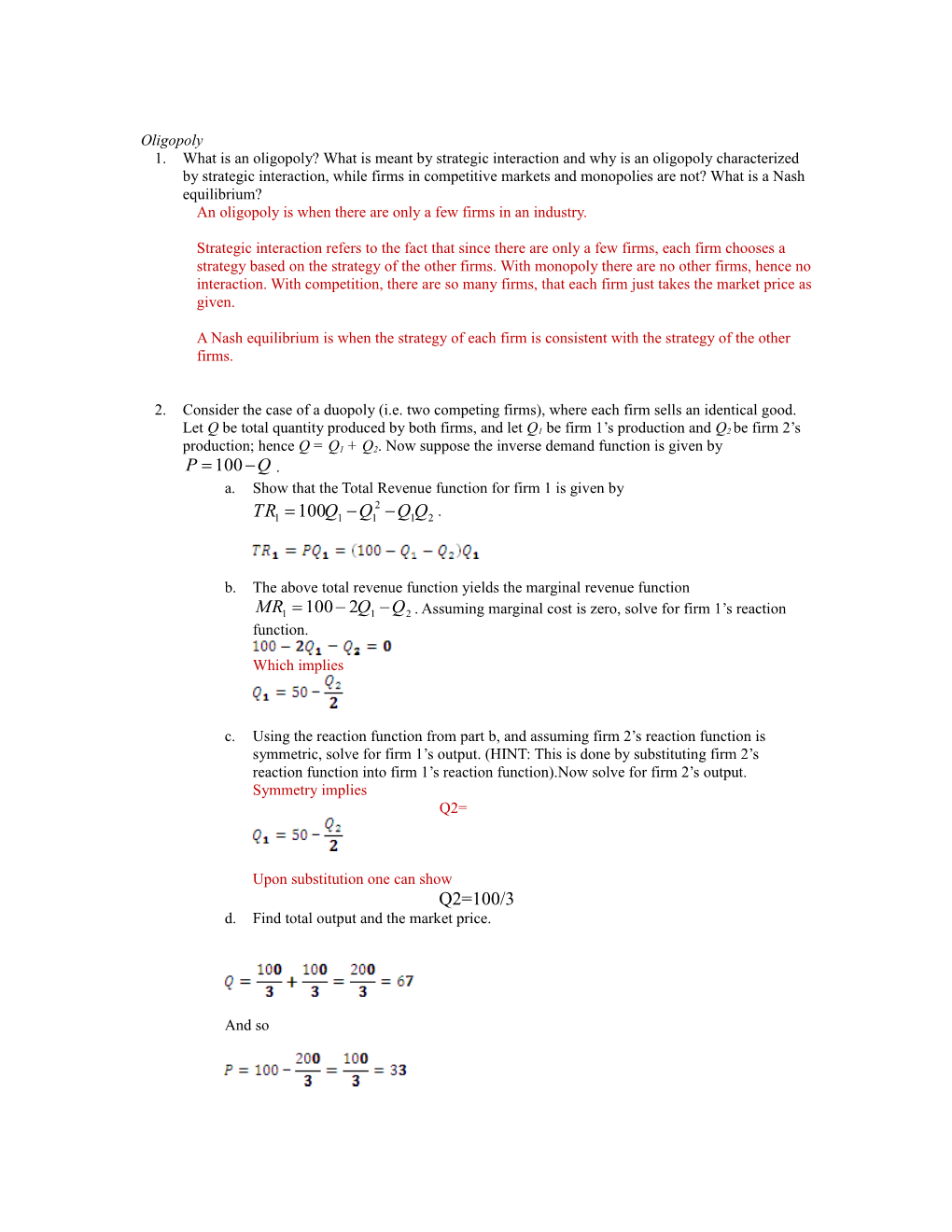# An Oligopoly Is When There Are Only a Few Firms in an IndustryOligopoly

1. What is an oligopoly? What is meant by strategic interaction and why is an oligopoly characterized by strategic interaction, while firms in competitive markets and monopolies are not? What is a Nash equilibrium?

An oligopoly is when there are only a few firms in an industry.

Strategic interaction refers to the fact that since there are only a few firms, each firm chooses a strategy based on the strategy of the other firms. With monopoly there are no other firms, hence no interaction. With competition, there are so many firms, that each firm just takes the market price as given.

A Nash equilibrium is when the strategy of each firm is consistent with the strategy of the other firms.

1. Consider the case of a duopoly (i.e. two competing firms), where each firm sells an identical good. Let Q be total quantity produced by both firms, and let Q1be firm 1’s production and Q2 be firm 2’s production; hence Q = Q1 + Q2. Now suppose the inverse demand function is given by.
2. Show that the Total Revenue function for firm 1 is given by .
1. The above total revenue function yields the marginal revenue function

. Assuming marginal cost is zero, solve for firm 1’s reaction function.

Which implies

1. Using the reaction function from part b, and assuming firm 2’s reaction function is symmetric, solve for firm 1’s output. (HINT: This is done by substituting firm 2’s reaction function into firm 1’s reaction function).Now solve for firm 2’s output.

Symmetry implies

Q2=

Upon substitution one can show

Q2=100/3

1. Find total output and the market price.

And so

Now suppose the firms colluded and behaved as a monopolist. Total revenue is then given by

TR = PQ = (100-Q)Q = 100Q – Q2. This implies the marginal revenue for a monopolist is MR = 100 – 2Q.

1. Find the monopolist quantity and price,

Setting MR = MC, we have

Which implies

By the pricing equation we then have

1. Using the fact that P = MC in equilibrium, find the competitive price and quantity.

So Q = 100, and P = 0/

1. Compare the competitive, monopoly, and oligopoly quantity. Would you say that an oligopoly is closer to competition or monopoly?

Competition:QC = 100

Duopoly:Q2 = 67

Monopoly:QM = 50

Hence two firms causes the market to move closer to the competitive outcome.

1. Consider a duopoly of the two firms, A and B. Suppose the two firms have an agreement to collude and behave as a single monopolist, but either firm may choose to break the agreement. The payoffs to the actions are given below:
• If both firms keep the collusive agreement, then each will have profits of 70.
• If one firm keeps the agreement, and the other firms breaks the agreement, the firm that keeps the agreement has profits of 40, and the one that breaks the agreement has profits of 100.
• If both firms break the agreement they each have profits of 50.
1. Set this up in a payoff matrix and identify the Nash equilibrium.

B’s Decison
Collude / Do Not Collude
A’s Decision / Collude / 70
70 / 40
100
Do Not Collude / 100
40 / 50
50

No matter what B does, A will not collude. No matter what A does, B will not collude. Hence the Nash equilibrium is where both players do not collude.

1. Explain how the Nash equilibrium will change if this is a repeated game.

In a repeated game, if one person does not collude the gain 30 in one period. But in future periods, they will be stuck at the NO Collude outcome, in which they lose 20. So there is a one-time gain of 30 at a cost of a loss of 20 every period after. Hence both players will choose to collude.